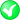#Boost C++ Libraries

...one of the most highly regarded and expertly designed C++ library projects in the world.

#### equals

Checks if a geometry are spatially equal.

###### Description

The free function equals checks if the first geometry is spatially equal the second geometry. Spatially equal means that the same point set is included. A box can therefore be spatially equal to a ring or a polygon, or a linestring can be spatially equal to a multi-linestring or a segment. This only theoretically, not all combinations are implemented yet.

###### Synopsis

```template<typename Geometry1, typename Geometry2>
bool equals(Geometry1 const & geometry1, Geometry2 const & geometry2)```

###### Parameters

Type

Concept

Name

Description

Geometry1 const &

Any type fulfilling a Geometry Concept

geometry1

A model of the specified concept

Geometry2 const &

Any type fulfilling a Geometry Concept

geometry2

A model of the specified concept

###### Returns

Returns true if two geometries are spatially equal

Either

`#include <boost/geometry/geometry.hpp>`

Or

`#include <boost/geometry/algorithms/equals.hpp>`

###### Conformance

The function equals implements function Equals from the OGC Simple Feature Specification.

###### Supported geometries

Point

Segment

Box

Linestring

Ring

Polygon

MultiPoint

MultiLinestring

MultiPolygon

PointSegmentBoxLinestringRingPolygonMultiPointMultiLinestringMultiPolygonLinear

###### Example

Shows the predicate equals, which returns true if two geometries are spatially equal

```#include <iostream>

#include <boost/geometry.hpp>
#include <boost/geometry/geometries/polygon.hpp>

BOOST_GEOMETRY_REGISTER_BOOST_TUPLE_CS(cs::cartesian)

#include <boost/assign.hpp>

int main()
{
using boost::assign::tuple_list_of;

typedef boost::tuple<int, int> point;

boost::geometry::model::polygon<point> poly1, poly2;
boost::geometry::exterior_ring(poly1) = tuple_list_of(0, 0)(0, 5)(5, 5)(5, 0)(0, 0);
boost::geometry::exterior_ring(poly2) = tuple_list_of(5, 0)(0, 0)(0, 5)(5, 5)(5, 0);

std::cout
<< "polygons are spatially "
<< (boost::geometry::equals(poly1, poly2) ? "equal" : "not equal")
<< std::endl;

boost::geometry::model::box<point> box;
boost::geometry::assign_values(box, 0, 0, 5, 5);

std::cout
<< "polygon and box are spatially "
<< (boost::geometry::equals(box, poly2) ? "equal" : "not equal")
<< std::endl;

return 0;
}
```

Output:

```polygons are spatially equal
polygon and box are spatially equal
```